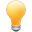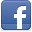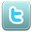Dart Closures Tutorial with Examples

View more Tutorials:Follow us on our fanpages to receive notifications every time there are new articles.FacebookTwitter

1- What is Closure?

In the Dart programming language, a Closure is a special function.
• Similar to a function, a Closure is a block of statements with parameters and can return a value or nothing.
• Unlike a function, a Closure has no name. However, you can identify a Closure through a variable.
Syntax:

// Closure syntax
(data_type1 arg1, data_type2 arg2, data_typeN argN) {
// Statements
}
// Declare a variable as an alias for Closure
var alias = (data_type1 arg1, data_type2 arg2, data_typeN argN) {
// Statements
};
Example: We declare a Closure with 1 parameter, and call it directly.
closure_ex1.dart

void main() {
// Create a Closure!
(String name) {
print('Hello \$name!');
};
// Create a Closure and call it directly!
(String name)  {
print('Bye \$name!');
}('Tom');
}
Output:

Bye Tom!
Example: Declare a variable as an identifier for a Closure:
closure_ex2.dart

void main() {
// Declare a variable as an alias for Closure
var sum = (int a, int b) {
return a + b;
};
// Call a closure.
var result = sum(100, 200); // 300
print('result = \$result');
}

2- Arrow Syntax

If the Closure's content consists of only one expression, you can write it more succinctly with the arrow syntax:

var alias1 =  (data_type1 arg1, data_type2 arg2, data_typeN argN) {
print('Something');
}
// Same as:
var alias1 =  (data_type1 arg1, data_type2 arg2, data_typeN argN) => print('Something');

var alias2 =  (data_type1 arg1, data_type2 arg2, data_typeN argN) {
return a_value;
}
// Same as:
var alias2 =  (data_type1 arg1, data_type2 arg2, data_typeN argN)  => a_value;
Example:
closure_arrow_ex1.dart

void main() {
// Create a Closure
var sum = (int a, int b) => a + b;

var result = sum(100, 200); // Call a closure.
print('result = \$result');

// Create a Closure
var sayHello = (String name) => print('Hello \$name');
sayHello('Tom'); // Call a closure.
}
Output:

result = 300
Hello Tom

3- Function Class

In the Dart language, a Closure is an object of the Function class, so you can declare a Closure with the following syntax:
Syntax:

Function(type1 arg1, type2 arg2, typeN argN) func = (type1 arg1, type2 arg2, typeN argN) {
// Statements
};
// Or:
return_type Function(type1 arg1, type2 arg2, typeN argN) func;

func = (type1 arg1, type2 arg2, typeN argN) {
// Statements
};
Example:
closure_func_ex1.dart

void main() {
// Function Type: (int, int) --> (int)
Function(int a, int b) sum = (int a, int b) {
return a + b;
};
// Function Type: (int, int) --> (int)
int Function(int a, int b) minus;
minus = (int a, int b) => a - b;

var result1 = sum(100, 200); // 300
print('result1 = \$result1');

var result2 = minus(100, 200); // -100
print('result2 = \$result2');
}

4- Closure in parameter

Closure can appear as a parameter of another function, closure, method, or constructor.

// A Function to calculate Tax.
// It accepts 2 arguments:
//  1 - int amount
//  2 - Closure
void printTaxAmount(int amount, double Function(int amount) taxFormula) {
var tax = taxFormula(amount);
print('tax = \$tax');
}

// Same as:
void printTaxAmount(int amount, double taxFormula(int amount)) {
var tax = taxFormula(amount);
print('tax = \$tax');
}
Example:
closure_in_args_ex1.dart

// A Function to calculate Tax.
// It accepts 2 arguments:
//  1 - int amount
//  2 - Closure
void printTaxAmount(int amount, double Function(int amount) taxFormula) {
var tax = taxFormula(amount);
print('tax = \$tax');
}
void main() {
// Create a Closure (Tax calucation formula of US)
var usTaxFormula = (int amount) {
return 10 * amount / 100; // return a double type.
};
// Create a Closure (Tax calucation formula of Vietnam)
var vnTaxFormula = (int amount) {
return 5 * amount / 100; // return a double type.
};
const amount = 1000;
printTaxAmount(amount, usTaxFormula); // 100.0
printTaxAmount(amount, vnTaxFormula); // 50.0
}
Output:

tax = 100.0
tax = 50.0
List.generate Example:
The List.generate constructor of the List class has a Closure as a parameter. Let's see an example of how to use this constructor.
List.generate Constructor

external factory List.generate(int length, E generator(int index), {bool growable = true});
Example:
closure_in_args_ex3.dart

// List.generate Constructor:
// external factory List.generate(int length, E generator(int index), {bool growable = true});
void main() {
// (int index) --> (String)
var generator = (int index) {
return 'Value ' + index.toString();
};
// Create a List with 5 elements:
List<String> myList = List.generate(5, generator, growable: true);
print(myList); // [Value 0, Value 1, Value 2, Value 3, Value 4]
}
Output:

[Value 0, Value 1, Value 2, Value 3, Value 4]

View more Tutorials:

Maybe you are interested

These are online courses outside the o7planning website that we introduced, which may include free or discounted courses.

•Google DART Programming for Android- BOOTCAMP
•The Complete 2020 Flutter Development Bootcamp with Dart
•Dart 2 Complete Bootcamp - Go Hero from Zero in Dart Flutter
•Understand Concepts of DART Programming quickly and easily!
•Learning Dart: Become more productive with Dart
•AngularDart - Build Dynamic Web Apps with Angular & Dart
•Dart Masterclass Programming Course: iOS/Android Bible
•Dart Programming from Beginner to Expert
•Flutter & Dart - The Complete Guide [2020 Edition]
•Beginning Web Components with Dart
•Learn Dart Programming for Flutter
•Master Flutter - Learn Dart & Flutter by Developing 20 Apps
•Dart 2 Programming Bootcamp For Complete Beginners
•Dart and Flutter: The Complete Developer's Guide
•Complete Dart programming
•Flutter & Dart: A Complete Showcase Mobile App™
•Google DART Programming HANDS-ON with PYTHON File Handling
•Introducing Dart
•Flutter & Dart - The Complete Flutter App Development Course
•Learn Dart 2 in 90 minutes
•Flutter & Dart Development For Building iOS and Android Apps
•AWS Mobile with Google Dart
•The Complete Flutter UI Masterclass | iOS & Android in Dart
•Dart & Flutter: The Complete Mobile Apps Development Course
•Dart and Flutter From Zero to Hero - Practical Dev Bootcamp